# How to Show the Formula Bar in Excel & Google Sheets

This tutorial demonstrates how to show the formula bar in Excel and Google Sheets.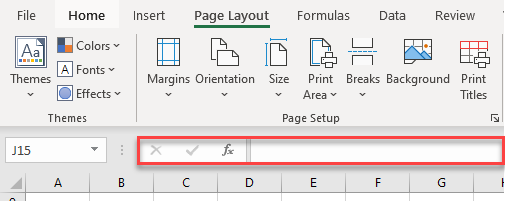## Show the Formula Bar

The picture above shows the formula bar in Excel. It’s possible to show and hide this part of the display, depending on your needs.

### Use the Ribbon

To show the formula bar using the Ribbon, follow these steps:

1. In the Ribbon, go to the View tab.
2. Check Formula Bar option.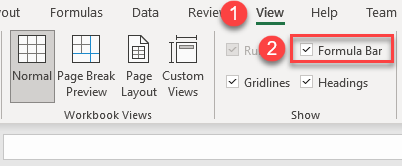Now you can see the formula bar.Unchecking the Formula Bar box shown in Step 2 would hide it again.

### Use Excel Options

Another way to show formula bar is to use Excel Options.

1. Click on the File tab in the Ribbon.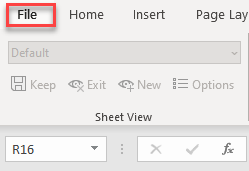1. From the drop-down menu, choose Options.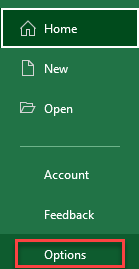1. Click on Advanced, scroll down to Display, and check the Show formula bar option.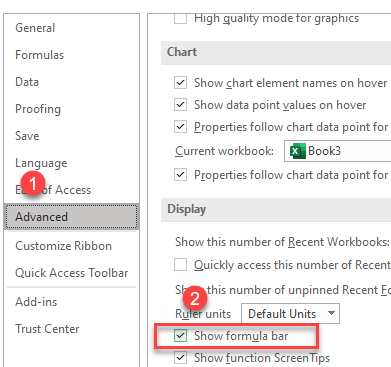The result is the same as with the method above: The formula bar is shown in Excel.Unchecking the Show formula bar box shown in Step 3 would hide the formula bar.

Note: You can also turn the formula bar on and off with VBA.

## Show Formula Bar in Google Sheets

You can also show the formula bar in Google Sheets.

1. From the Menu, go to View.
2. Make sure Formula bar is checked.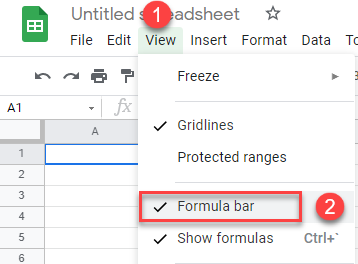Now you can see the formula bar.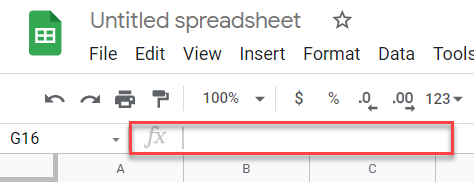Deselecting the Formula bar option shown in Step 2 would hide the formula bar.